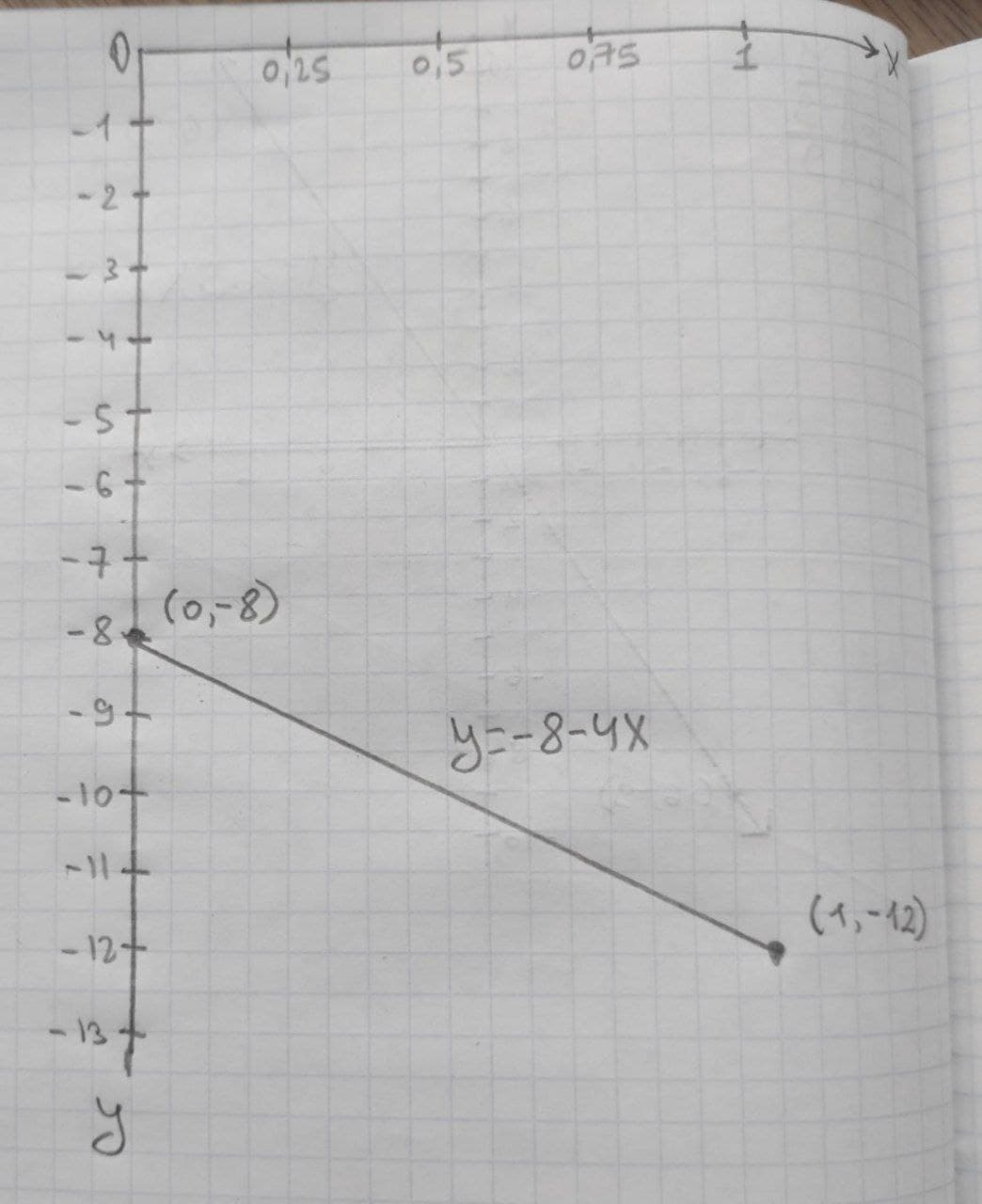# Given linear equation y = -8-4x a. find the y-intercept and slope. b. determine whether the line slopes upward, slopes downward, or is horizontal, without graphing the equation. c. use two points to graph the equation.defazajx 2020-12-21 Answered
Given linear equation y = -8-4x
a. find the y-intercept and slope.
b. determine whether the line slopes upward, slopes downward, or is horizontal, without graphing the equation.
c. use two points to graph the equation.
You can still ask an expert for help

• Questions are typically answered in as fast as 30 minutes

Solve your problem for the price of one coffee

• Math expert for every subject
• Pay only if we can solve itElberte
(a) Given linear equation is y= -8-4x.
Thus,
Intercept = -8
Slope = -4
(b) The slope is upward if slope > 0.
The slope is downward if slope < 0.
The slope is horizontal if slope = 0.
Here, slope of the line is -4, therefore slope is downward.
(c) The line is y= -8-4x.
If x=0 then y=-8.
If x=1 then y=-12.
Consider the points (0,-8) and (1,-12)
The graph for the line with the given equation with the obtained points is given below: